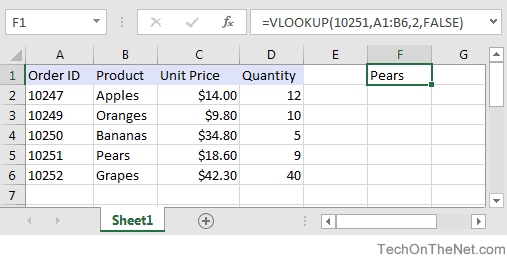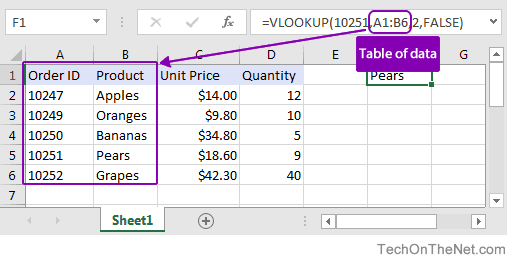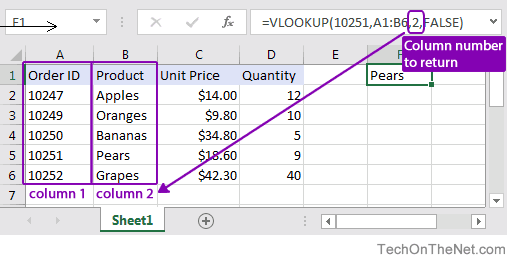# How to use the VLOOKUP FunctionA lot of people try to use Excel as a database. While it can work as a database, some of the tasks that would be very easy in a database program are fairly complex in Excel. One of these tasks is matching two lists based on a common field; this can be easily accomplished using Excel VLOOKUP. You will find the function VLOOKUP to be extremely useful so check out an example of when and how to use this function below.

This Excel tutorial explains how to use the VLOOKUP function with syntax and examples.

## Description

The VLOOKUP function performs a vertical lookup by searching for a value in the first column of a table and returning the value in the same row in the index_number position.

The VLOOKUP function is a built-in function in Excel that is categorized as a Lookup/Reference Function. It can be used as a worksheet function (WS) in Excel. As a worksheet function, the VLOOKUP function can be entered as part of a formula in a cell of a worksheet.The VLOOKUP function is actually quite easy to use once you understand how it works! .

## Syntax

The syntax for the VLOOKUP function in Microsoft Excel is:

`VLOOKUP( value, table, index_number, [approximate_match] )`

### Parameters or Arguments

value
The value to search for in the first column of the table.
table
Two or more columns of data that is sorted in ascending order.
index_number
The column number in table from which the matching value must be returned. The first column is 1.
approximate_match
Optional. Enter FALSE to find an exact match. Enter TRUE to find an approximate match. If this parameter is omitted, TRUE is the default.

## Note

• If you specify FALSE for the approximate_match parameter and no exact match is found, then the VLOOKUP function will return #N/A.
• If you specify TRUE for the approximate_match parameter and no exact match is found, then the next smaller value is returned.
• If index_number is less than 1, the VLOOKUP function will return #VALUE!.
• If index_number is greater than the number of columns in table, the VLOOKUP function will return #REF!.
• See also the HLOOKUP function to perform a horizontal lookup.

## Applies To

• Excel 2016, Excel 2013, Excel 2011 for Mac, Excel 2010, Excel 2007, Excel 2003, Excel XP, Excel 2000

## Type of Function

• Worksheet function (WS)

## Example (as Worksheet Function)

Let’s explore how to use VLOOKUP as a worksheet function in Microsoft Excel.Based on the Excel spreadsheet above, the following VLOOKUP examples would return:

```=VLOOKUP(10251, A1:B6, 2, FALSE)
Result: "Pears"   'Returns value in 2nd column

=VLOOKUP(10251, A1:C6, 3, FALSE)
Result: \$18.60    'Returns value in 3rd column

=VLOOKUP(10251, A1:D6, 4, FALSE)
Result: 9         'Returns value in 4th column

=VLOOKUP(10248, A1:B6, 2, FALSE)
Result: #N/A      'Returns #N/A error (no exact match)

=VLOOKUP(10248, A1:B6, 2, TRUE)
Result: "Apples"  'Returns an approximate match```

Now, let’s look at the example `=VLOOKUP(10251, A1:B6, 2, FALSE)` that returns a value of “Pears” and take a closer look why.

### First Parameter

The first parameter in the VLOOKUP function is the value to search for in the table of data.In this example, the first parameter is 10251. This is the value that the VLOOKUP will search for in the first column of the table of data. Because it is a numeric value, you can just enter the number. But if the search value was text, you would need to put it in double quotes, for example:

`=VLOOKUP("10251", A1:B6, 2, FALSE)`

### Second Parameter

The second parameter in the VLOOKUP function is the table or the source of data where the vertical lookup should be performed.In this example, the second parameter is A1:B6 which gives us two columns to data to use in the vertical lookup – A1:A6 and B1:B6. The first column in the range (A1:A6) is used to search for the Order value of 10251. The second column in the range (B1:B6) contains the value to return which is the Product value.

### Third Parameter

The third parameter is the position number in the table where the return data can be found. A value of 1 indicates the first column in the table. The second column is 2, and so on.In this example, the third parameter is 2. This means that the second column in the table is where we will find the value to return. Since the table range is set to A1:B6, the return value will be in the second column somewhere in the range B1:B6.

### Fourth Parameter

Finally and most importantly is the fourth or last parameter in the VLOOKUP. This parameter determines whether you are looking for an exact match or approximate match.In this example, the fourth parameter is FALSE. A parameter of FALSE means that VLOOKUP is looking for an EXACT match for the value of 10251. A parameter of TRUE means that a “close” match will be returned. Since the VLOOKUP is able to find the value of 10251 in the range A1:A6, it returns the corresponding value from B1:B6 which is Pears.

### Exact Match vs. Approximate Match

To find an exact match, use FALSE as the final parameter. To find an approximate match, use TRUE as the final parameter.

Let’s lookup a value that does not exist in our data to demonstrate the importance of this parameter!

#### Exact Match

Use FALSE to find an exact match:

```=VLOOKUP(10248, A1:B6, 2, FALSE)
Result: #N/A```

If no exact match is found, #N/A is returned.

#### Approximate Match

Use TRUE to find an approximate match:

```=VLOOKUP(10248, A1:B6, 2, TRUE)
Result: "Apples"```

If no match is found, it returns the next smaller value which in this case is “Apples”.

### VLOOKUP from Another Sheet

You can use the VLOOKUP to lookup a value when the table is on another sheet. Let’s modify our example above and assume that the table is in a different Sheet called Sheet2 in the range A1:B6.

We could rewrite our original example where we lookup the value 10251 as follows:

`=VLOOKUP(10251, Sheet2!A1:B6, 2, FALSE)`

By preceding the table range with the sheet name and an exclamation mark, we can update our VLOOKUP to reference a table on another sheet.

### VLOOKUP from Another Sheet with Spaces in Sheet Name

Let’s throw in one more complication, what happens if your Sheet name contains spaces, then you will need to change the formula further.

Let’s assume that the table is on a Sheet called “Test Sheet” in the range A1:B6, now we need to wrap the Sheet name in single quotes as follows:

`=VLOOKUP(10251, 'Test Sheet'!A1:B6, 2, FALSE)`

By placing the sheet name within single quotes, we can handle a sheet name with spaces in the VLOOKUP function.

### VLOOKUP from Another Workbook

You can use the VLOOKUP to lookup a value in another workbook. For example, if you wanted to have the table portion of the VLOOKUP formula be from an external workbook, we could try the following formula:

`=VLOOKUP(10251, 'C:\[data.xlsx]Sheet1'!\$A\$1:\$B\$6, 2, FALSE)`

This would look for the value 10251 in the file C:\data.xlxs in Sheet 1 where the table data is found in the range \$A\$1:\$B\$6.

### Why use Absolute Referencing?

Now it is important for us to cover one more mistake that is commonly made. When people use the VLOOKUP function, they commonly use relative referencing for the table range like we did in some of our examples above. This will return the right answer, but what happens when you copy the formula to another cell? The table range will be adjusted by Excel and change relative to where you paste the new formula. Let’s explain further…

So if you had the following formula in cell G1:

`=VLOOKUP(10251, A1:B6, 2, FALSE)`

And then you copied this formula from cell G1 to cell H2, it would modify the VLOOKUP formula to this:

`=VLOOKUP(10251, B2:C7, 2, FALSE)`

Since your table is found in the range A1:B6 and not B2:C7, your formula would return erroneous results in cell H2. To ensure that your range is not changed, try referencing your table range using absolute referencing as follows:

`=VLOOKUP(10251, \$A\$1:\$B\$6, 2, FALSE)`

Now if you copy this formula to another cell, your table range will remain \$A\$1:\$B\$6.

### How to Handle #N/A Errors

Next, let’s look at how to handle instances where the VLOOKUP function does not find a match and returns the #N/A error. In most cases, you don’t want to see #N/A but would rather display a more user-friendly result.

For example, if you had the following formula:

`=VLOOKUP(10248, \$A\$1:\$B\$6, 2, FALSE)`

Instead of displaying #N/A error if you do not find a match, you could return the value “Not Found”. To do this, you could modify your VLOOKUP formula as follows:

`=IF(ISNA(VLOOKUP(10248, \$A\$1:\$B\$6, 2, FALSE)), "Not Found", VLOOKUP(10248, \$A\$1:\$B\$6, 2, FALSE))`

This new formula will use the ISNA function to test if the VLOOKUP returns a #N/A error. If the VLOOKUP returns #N/A, then the formula will output “Not Found”. Otherwise, it will perform the VLOOKUP as before.

This is a great way to spruce up your spreadsheet so that you don’t see traditional Excel errors.